Courses

# Mohr's Circle for Moments of Inertia Mechanical Engineering Notes | EduRev

## Mechanical Engineering : Mohr's Circle for Moments of Inertia Mechanical Engineering Notes | EduRev

The document Mohr's Circle for Moments of Inertia Mechanical Engineering Notes | EduRev is a part of the Mechanical Engineering Course Engineering Mechanics - Notes, Videos, MCQs & PPTs.
All you need of Mechanical Engineering at this link: Mechanical Engineering

Principal Axes and Principal Moments of Inertia

Squaring Eqs. 10-9a and 10-9c and adding, it is found that;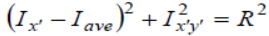where: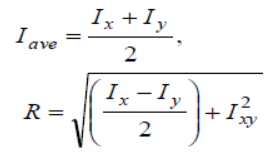• At the points A and B, Ix’y’ = 0 and Ix’ is a maximum and minimum, respectively.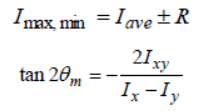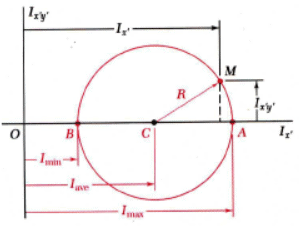• The equation for qm defines two angles, 90o apart which correspond to the principal axes of the area about O.
• Imax and Imin are the principal moments of inertia of the area about O.

Mohr’s Circle for Moments and Products of Inertia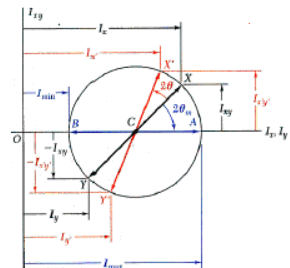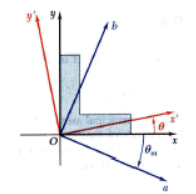• The moments and product of inertia for an area are plotted as shown and used to construct Mohr’s circle,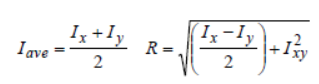• Mohr’s circle may be used to graphically or analytically determine the moments and product of inertia for any other rectangular axes including the principal axes and principal moments and products of inertia.

Sample Problem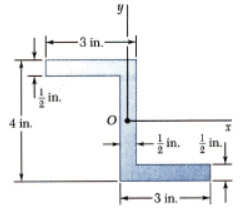For the section shown, the moments of inertia with respect to the xand yaxes are Ix= 10.38 in4and Iy= 6.97 in4.

Determine (a) the orientation of the principal axes of the section about O,and (b) the values of the principal moments of inertia about O.

Solution:

•Compute the product of inertia with respect to the xyaxes by dividing the section into three rectangles and applying the parallel axis theorem to each.
•Determine the orientation of the principal axes (Eq. 9.25) and the principal moments of inertia (Eq. 9. 27).

Sample Problem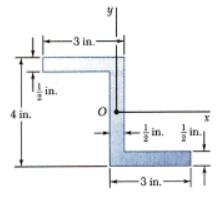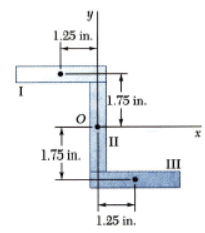Solution:

• Compute the product of inertia with respect to the xy axes by dividing the section into three rectangles.

Apply the parallel axis theorem to each rectangle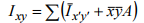Note that the product of inertia with respect to centroidal axes parallel to the xy axes is zero for each rectangle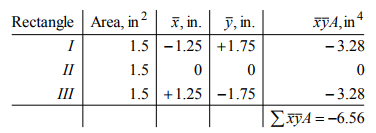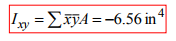Sample Problem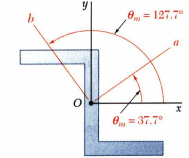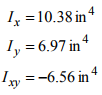• Determine the orientation of the principal axes (Eq. 9.25) and the principal moments of inertia (Eq. 9. 27).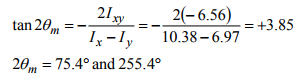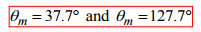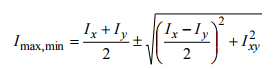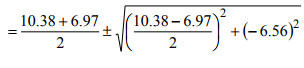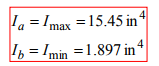Sample Problem 10.8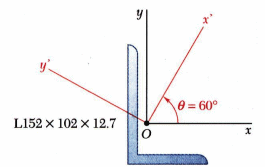The moments and product of inertia with respect to the x and y axes are Ix = 7.24x106 mm4 , Iy = 2.61x106 mm4 , and Ixy = -2.54x106 mm4.

Using Mohr’s circle, determine (a) the principal axes about O, (b) the values of the principal moments about O, and (c) the values of the moments and product of inertia about the x’ and y’ axes

Solution:

• Plot the points (Ix , Ixy ) and (Iy ,-Ixy ). Construct Mohr’s circle based on the circle diameter between the points.
• Based on the circle, determine the orientation of the principal axes and the principal moments of inertia.
• Based on the circle, evaluate the moments and product of inertia with respect to the x’y’ axes.

Sample Problem 10.8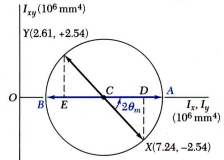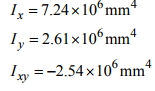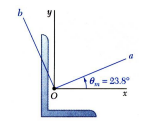Solution:

• Plot the points (Ix , Ixy ) and (Iy ,-Ixy ). Construct Mohr’s circle based on the circle diameter between the points.
•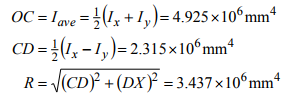• Based on the circle, determine the orientation of the principal axes and the principal moments of inertia.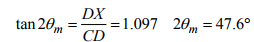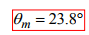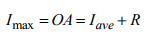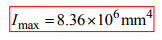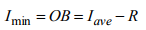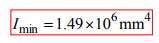Sample Problem 10.8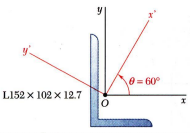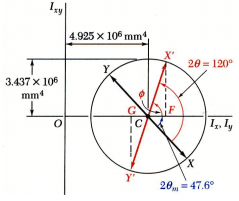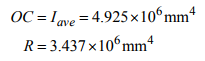• Based on the circle, evaluate the moments and product of inertia with respect to the x’y’ axes

The points X’ and Y’ corresponding to the x’ and y’ axes are obtained by rotating CX and CY counterclockwise through an angle Q = 2(60o ) = 120o . The angle that CX’ forms with the x’ axes is φ = 120o - 47.6o = 72.4o .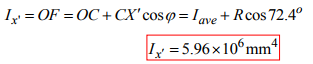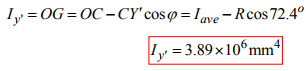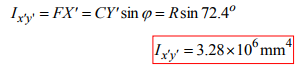Offer running on EduRev: Apply code STAYHOME200 to get INR 200 off on our premium plan EduRev Infinity!

,

,

,

,

,

,

,

,

,

,

,

,

,

,

,

,

,

,

,

,

,

;